### Write a C program to find the Simple Interest. Take input for principle amount, rate of interest and time from terminal

Simple Interest is the money paid by the borrower to the lender, based on the Principle Amount, Interest Rate and the Time Period.

Simple Interest is calculated by, SI= P * T * R / 100 formula.

Where, P is the Principle Amount.
T is the Time Period.
R is the Interest Rate.

## Definition of 'Simple Interest' from WEB

A quick method of calculating the interest charge on a loan. Simple interest is determined by multiplying the interest rate by the principal by the number of periods.
Simple Interest = P x I x N

Where:

P is the loan amount
I is the interest rate
N is the duration of the loan, using number of periods

## Explanation of 'Simple Interest'

Simple interest is called simple because it ignores the effects of compounding. The interest charge is always based on the original principal, so interest on interest is not included.  This method may be used to find the interest charge for short-term loans, where ignoring compounding is less of an issue.
Source Code:

#include<stdio.h>
main()
{
float jama,suderhar,somoy;
float sud=0;
scanf("%f",&jama);
printf("\nEnter Your Rate of Interest: ");
scanf("%f",&suderhar);
printf("\nEnter The Time Period: ");
scanf("%f",&somoy);
suderhar=suderhar/100;
sud=jama*suderhar*somoy;
printf("The calculated interest is: %f",sud);
return 0;
}
<< Go to Index Page >>

1.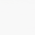#include
#include
void main()
{
int p,t;
float r;
float SI;
printf("\nEnter the Principle Amount, Terms, Rate of Interest :");
scanf("%d%d%f",&p,&t,&r);
printf("\nThe Simple Interest for the Principle \nAmount %d for %d Years with an Interest of %0.2f",p,t,r);
SI=((p * t * r) / 100);
printf("\n is %5.0f.",SI);

getch();
}

2.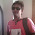printf("\n is %5.0f.",SI);

I think here some problem for out put show

3.#include
main()
{
int p,t;
float r;
float SI;
printf("\nEnter the Principle Amount,Time of period, Rate of Interest respectively:");
scanf("%d%d%f",&p,&t,&r);
SI=((p * t * r) / 100);
printf("\nInterest is: %.2f",SI);
getch();
}

modify by Ashraf

4.I am so happy to see all above discussion. Keep it up.

5.I am so happy to see all above discussion. Keep it up.

6.sir,i want to make the program in real use...try to make prompt program...here it is

#include
#include

main()
{
float si,p,i,t;
char ch;
si:
scanf("%f",&p);
printf("enter your time(in year) : ");
scanf("%f",&t);
printf("enter your interst rate : ");
scanf("%f",&i);
printf("you have entered,principle=%f\n time=%f year\n interst rate=%f\n",p,t,i);
printf("if your entered data is correct press 'y' , otherwise press 'n' : ");
scanf("%s",&ch);
if (ch=='y' || ch=='Y')
{
si=(p*i*t)/100;
printf("your simple interest is = %f",si);
}
else if (ch=='n'||ch=='N')
goto si;
getch();
}

7.Great....That's real programmer.

8.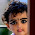#include
#include

main()
{
float si,p,i,t;
char ch;
si:
scanf("%f",&p);
printf("enter your time(in year) : ");
scanf("%f",&t);
printf("enter your interst rate : ");
scanf("%f",&i);
printf("you have entered,principle=%f\n time=%f year\n interst rate=%f\n",p,t,i);
printf("if your entered data is correct press 'y' , otherwise press 'n' : ");
scanf("%s",&ch);
if (ch=='y' || ch=='Y')
{
si=(p*i*t)/100;
printf("your simple interest is = %f",si);
}
else if (ch=='n'||ch=='N')
goto si;
getch();
}

9.#include
#include
int main()
{
int num1,num2;
float res;
char opt;
calc:
scanf("%d%c%d",&num1,&opt,&num2);

switch(opt)
{
case '+':res=num1+num2;
printf("=%f\n",res);
break;
case '-':res=num1-num2;
printf("=%f\n",res);
break;
case '*': res=num1*num2;
printf("=%f\n",res);
break;
case '%':res=num1%num2;
printf("=%f\n",res);
break;
case '/':(float)res=(float)num1/(float)num2;
printf("=%f\n",res);
break;
default : printf("error ! enter valid sign\n");
break;
}
goto calc;
getch();
return 0;
}

10.i am so happy to do it !

11.Very informative post indeed.. being enrolled in http://www.wiziq.com/course/6314-learn-c-programming-language-low-priced-student-edition was looking for such articles online to assist me.. and your post helped me a lot

12.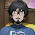#include
int main()
{
float a,b,c,si;
printf("\nEnter the amount: ");
scanf("%f",&a);
printf("\nRate: ");
scanf("%f",&b);
printf("\nTime: ");
scanf("%f",&c);
si=(a*b*c)/100;
printf("\nThe simple interest=%f",si);
}

13.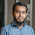#include
#include
main(){
int jama,suderhar,somoy;
int sud;
suderhar=suderhar/100;
scanf("%d",&jama);
scanf("%d",&suderhar);
scanf("%d",&somoy);
sud=jama*suderhar*somoy;
printf("%d",sud);
return 0;
}

14.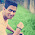Md.Hasmot Hossen
ID:201421066

#include
#include
class interest
{
int n;
float rate,p;
public:
void get()
{
cout<<“\nEnter principle Amount & no. of year: \n”;
cin>>p>>n;
}
void cal(float rate)
{
float si;
si=(p*n*rate)/100;
cout<<“\n\nSimple Interest is: “<<si;
}
};
void main()
{
interest i;
clrscr();
i.get();
i.cal(4.5);
getch();
}

15.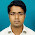#include
main()
{
int x,y;
float z;
float SI;
printf("\nEnter the Principle Amount,Time period, Rate of Interest respectively:");
scanf("%d%d%f",&x,&y,&z);
SI=((x * y * z) / 100);
printf("\nInterest is: %.2f",SI);
getch();
}

16.//*WAP a Program to find Simple Interest using constructor*//

#include
using namespace std;
class interest
{
public:
int p,t,r;
int si;
interest();
~interest();
void S_interest();
};
interest::interest()
{
cout<<" ";

}
interest::~interest()
{
cout<<"destructing"<>p;
cout<<"Enter the Time:"<>t;
cout<<"Enter the Rate:"<>r;
si=(p*t*r)/100;
cout<<"The total simple interest is:"<<endl;
cout<<si<<endl;
}

int main()
{
interest ob;
ob.S_interest();
return 0;

}

17.#include
using namespace std;
class interest
{
public:
int p;
float t,r;
interest();
~interest();
void intrst();
};
interest::interest()
{
cout<<"Enter Principle Amount, Time(year) And Rate:";
cin>>p>>t>>r;
}
interest::~interest()
{

}
void interest::intrst()
{
float inter;
inter=(p*t*r)/100;
cout<<endl<<"Interest is: "<<inter<<"Tk";
}
main()
{
interest in;
in.intrst();
}

18.#include
using namespace std;
class arrmani
{
public:
int len,i;
int a;//Here is size but it is Fakibaji with compiler
arrmani();
~arrmani();
void leveling();
void ev_oddchk();
void show();
void finder();

protected:
int o,e,osum,esum,allsum,count,p,q;
float avg;
};

arrmani::arrmani()
{
cout<<"Array size?";
cin>>len;
cout<<"Enter array values: "<>a[i];

}

arrmani::~arrmani()
{

}

void arrmani::leveling()
{
int b=5;
while(b!=0)
{
b=0;
int j=0;
for(i=1;i=a[i])
{
p=a[j];
a[j]=a[i];
a[i]=p;
b=1;
}
j++;
}

}
cout<<"Leveled array is: ";
for(i=0;ia[i])
{
q=a[i];
}

}
}

void arrmani::show()
{
cout<<"Number of value is: "<<count<<endl;
cout<<"Total value is: "<<allsum<<endl;
cout<<"Average value is: "<<avg<<endl;
cout<<"Number of even value is: "<<e<<endl;
cout<<"Summation of even value is: "<<esum<<endl;
cout<<"Number of odd value is: "<<o<<endl;
cout<<"Summation of odd value is: "<<osum<<endl;
cout<<"largest value is : "<<p<<endl;
cout<<"Smalest value is : "<<q<<endl;

}

int main()
{
arrmani an;
an.leveling();
an.ev_oddchk();
an.finder();
an.show();
return 0;}

19.#include
using namespace std;
class arrmani
{
public:
int len,i;
int a;//Here is size but it is Fakibaji with compiler
arrmani();
~arrmani();
void leveling();
void ev_oddchk();
void show();
void finder();

protected:
int o,e,osum,esum,allsum,count,p,q;
float avg;
};

arrmani::arrmani()
{
cout<<"Array size?";
cin>>len;
cout<<"Enter array values: "<>a[i];

}

arrmani::~arrmani()
{

}

void arrmani::leveling()
{
int b=5;
while(b!=0)
{
b=0;
int j=0;
for(i=1;i=a[i])
{
p=a[j];
a[j]=a[i];
a[i]=p;
b=1;
}
j++;
}

}
cout<<"Leveled array is: ";
for(i=0;ia[i])
{
q=a[i];
}

}
}

void arrmani::show()
{
cout<<"Number of value is: "<<count<<endl;
cout<<"Total value is: "<<allsum<<endl;
cout<<"Average value is: "<<avg<<endl;
cout<<"Number of even value is: "<<e<<endl;
cout<<"Summation of even value is: "<<esum<<endl;
cout<<"Number of odd value is: "<<o<<endl;
cout<<"Summation of odd value is: "<<osum<<endl;
cout<<"largest value is : "<<p<<endl;
cout<<"Smalest value is : "<<q<<endl;

}

int main()
{
arrmani an;
an.leveling();
an.ev_oddchk();
an.finder();
an.show();
return 0;}

20.#include
using namespace std;
int main()
{
int a,b;
cin>>a;
cin>>b;
cout<<a+a*b/100;
}

21.#include
using namespace std;

int main()
{
int period, principal;
float inrate, interest;

cout<<"What's the amount of your principal: ";
cin>>principal;

cout<>period;

cout<>inrate;
cout<<endl;

interest=period*principal*inrate;

cout<<"The total interest is: "<<interest;

return 0;
}

22.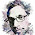Appel mahmud akib. Id:201510384

#include
using namespace std;
int main()
{
int p,t,r;
cout<<"Input principal amount: ";
cin>>p;
cout<<"Input interest rate: ";
cin>>r;
cout<<"Input time: ";
cin>>t;
cout<<endl<<(p*t*r)/100<<endl;

}

23.#include
using namespace std;
int main()
{
float far;
cout<<"Input Temperature in farenheit: ";
cin>>far;
cout<<"Your temperature in celcius:(7.0/9.0) * (far-32)";
}

24.#include
using namespace std;
int main()
{
int p,t,r;
cout<<"Input principal amount: ";
cin>>p;
cout<<"Input interest rate: ";
cin>>q;
cout<<"Input time: ";
cin>>t;
cout<<endl<<(p*q*r)/100<<endl;

}

25.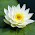using c++

#include

using namespace std;

float func(float money, float interest, int time)
{
return ((money*time*interest)/100);
}

int main()
{
float i, j;
int k;
cout << "Enter total ammount --: ";
cin >> i;
cout << "Enter interest rate in (%) --: ";
cin >> j;
cin >> k;
cout <<"The calculated interest is --: " << func(i, j, k);
return 0;
}

26.Using C++
#include
using namespace std;

int main()
{
int i,t,a,sum;

cout<<"Enter The amount";
cin >>a;
cout<<"Enter The interest";
cin >>i;
cout<<"Enter The time";
cin >>t;
sum =a*i*t/100;
cout<<a*t*i/100<<sum;

return 0;
}

27.using c++

#include
using namespace std;
int main()
{
int p,t,r;
cout<<"Input principal amount: ";
cin>>p;
cout<<"Input interest rate: ";
cin>>r;
cout<<"Input time: ";
cin>>t;
cout<<endl<<(p*t*r)/100<<endl;

}

28.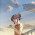#include
using namespace std;

int main()
{
double amount,interest,time, total;

cout <<"Enter the amount: "<< endl;
cin >>amount;
cout <<"Enter the interest: "<< endl;
cin >>interest;
cout <<"Enter the time: "<< endl;
cin >>time;
interest = interest/100;
total =(amount*interest*time);
cout <<"The total interest is: "<<total<< endl;
return 0;
}

id:201510992

29.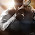#include

main()

{
int a,b,result ;
printf("Enter a: \n");
scanf("%d",&a);

printf("Enter b: \n");
scanf("%d",&b);

result = a*a+2*a*b+b*b;
printf("The result of: %d",result);

}

30.#include

main()

{
int a,b,result ;
printf("Enter a: \n");
scanf("%d",&a);

printf("Enter b: \n");
scanf("%d",&b);

result = a*a+2*a*b+b*b;
printf("The result of: %d",result);

}

31.#include

main()

{
int a,b,result ;
printf("Enter a: \n");
scanf("%d",&a);

printf("Enter b: \n");
scanf("%d",&b);

result = a*a+2*a*b+b*b;
printf("The result of: %d",result);

}

32.#include

main()

{
int a,b,result ;
printf("Enter a: \n");
scanf("%d",&a);

printf("Enter b: \n");
scanf("%d",&b);

result = a*a+2*a*b+b*b;
printf("The result of: %d",result);

}

33.#include

main()

{
int a,b,result ;
printf("Enter a: \n");
scanf("%d",&a);

printf("Enter b: \n");
scanf("%d",&b);

result = a*a+2*a*b+b*b;
printf("The result of: %d",result);

}

34.#include
main()
{
float jama,suderhar,somoy;
float sud=0;
scanf("%f",&jama);
printf("\nEnter Your Rate of Interest: ");
scanf("%f",&suderhar);
printf("\nEnter The Time Period: ");
scanf("%f",&somoy);
suderhar=suderhar/100;
sud=jama*suderhar*somoy;
printf("The calculated interest is: %f",sud);
return 0;
}

35.#include

main()

{
int a,b,result ;
printf("Enter a: \n");
scanf("%d",&a);

printf("Enter b: \n");
scanf("%d",&b);

result = a*a+2*a*b+b*b;
printf("The result of: %d",result);

}

36.#include
using namespace std;
int main()
{
float m,s,t,i=0;
cout<<"Enter Your Rate of Interest: " <>s;
cout<<"Enter The Time Period: "<>t;
s=s/100;
i=m*s*t;
cout<<"The calculated interest is: "<<i;
}

37.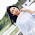#include
int main(){
int a,i,t,sum=0;
printf("enter the amount=");
scanf("%d",&a);
printf("enter the interest=");
scanf("%d",&i);
printf("enter the time=");
scanf("%d",&t);
sum+=a*i*t/100;
printf("%d",sum);
}

38.#include

main()

{
int a,b,result ;
printf("Enter a: \n");
scanf("%d",&a);

printf("Enter b: \n");
scanf("%d",&b);

result = a*a+2*a*b+b*b;
printf("The result of: %d",result);

}

39.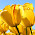Summer 2017
ID#201610042

Answer to the Question No. 2:

40.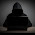https://www.dropbox.com/s/dfb2awx0euj1km3/Simple_Interest02.cpp?dl=0

41.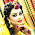42.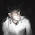43.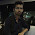ID : 201620195

44.45.id no:201610259
name: jahirul islam

46.47.48.49.50.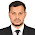51.ID 201610456
Name MD USUF MIA

52.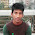ID:201520552

53.ID 201610456
MD USUF MIA

54.55.Id:201620318

56.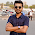https://www.dropbox.com/s/llfu5v0onalxasa/HW2.cpp?dl=0

57.https://www.dropbox.com/s/cq4sotgxib8k82q/HW2%20paramiter.cpp?dl=0

58.https://www.dropbox.com/s/eun02fve1t51a3g/HW2%20constructor.cpp?dl=0

59.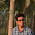https://www.dropbox.com/s/kul1hqh8c8i1emu/account.cpp?dl=0

60.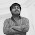Id= 201710494
https://www.dropbox.com/s/f9pp7byxe62mhis/prob%202.cpp?dl=0

61.62.ID : 201620128

63.Nusrat Sumaiya AshrafeJune 8, 2017 at 8:33 AM

ID: 201710950

64.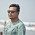https://www.dropbox.com/s/b0l64cd8y17q1ro/HW2.cpp?dl=0

65.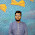66.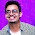67.ID:-201610058

68.#include
using namespace std;

//Editor: Md Rakibul Hasan Prince
// ID: 201720620

class interest
{
public:
double si,p,t,r;
interest()
{
cout<<"Enter the principle amount: ";
cin>>p;
cout<<"Enter the interest rate: ";
cin>>r;
cout<<"Enter the time period: ";
cin>>t;
}
double calc()
{
si=p*t*r/100;
return si;
}
~interest()
{
}
};
int main()
{
cout<<"Program To Find The Simple Interest\n";
interest ob;
cout<<ob.calc()<<"\n";
return 0;
}

69.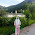Name:Sumsunnahar nawa
ID:201710090
Batch:54(CSE)

70.ID: 201710404

71.ID #201610288

72.ID #201610288

73.ID-201820298
58 BATCH

#include
int main()
{
float x,y,z,si;
printf("\nEnter the amount: ");
scanf("%f",&x);
printf("\nRate: ");
scanf("%f",&y);
printf("\nTime: ");
scanf("%f",&z);
si=(x*y*z)/100;
printf("\nThe simple interest=%f",si);
}

74.#include
int main()
{
float Loan, time, rate, SI;
printf("Enter Loan (amount): ");
scanf("%f", &Loan);

printf("Enter time: ");
scanf("%f", &time);

printf("Enter rate: ");
scanf("%f", &rate);

SI = (Loan * time * rate) / 100;

printf("Simple Interest = %f", SI);

return 0;
}

75.#include
int main()
{
float Loan, time, rate, SI;
printf("Enter Loan (amount): ");
scanf("%f", &Loan);

printf("Enter time: ");
scanf("%f", &time);

printf("Enter rate: ");
scanf("%f", &rate);

SI = (Loan * time * rate) / 100;

printf("Simple Interest = %f", SI);

return 0;
}

76.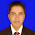ID: 201820244
Batch:58th
Section: B

#include
int main()
{
float taka,sud,time;
float sudd=0;
scanf("%f",&taka);
printf("\nEnter Your Rate of Interest: ");
scanf("%f",&sud);
printf("\nEnter The Time Period: ");
scanf("%f",&time);
sudd=sud/100;
sud=taka*sudd*time;
printf("The calculated interest is: %f",sud);
return 0;
}

77.ID#201830546

#include
using namespace std;
int main()
{
int p,t,r;
cout<<"Input principal amount: ";
cin>>p;
cout<<"Input interest rate: ";
cin>>r;
cout<<"Input time: ";
cin>>t;
cout<<endl<<(p*t*r)/100<<endl;

}

78.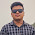Name : Firoj Ahmed
ID : 201820222
Batch : 58th

#include
using namespace std;

class SimpleI
{
// private variable
float P,T,R;
public:
float interest()
{
cout << "Enter Your Principal Amount: ";
cin>>P;
cout << "Enter Your Rate of Interest: ";
cin>>R;
cout << "Enter The Time Period: ";
cin>>T;

R = R/100;
return P*T*R;
}
};

int main()
{
SimpleI SI;

cout<<"The calculated interest is: "<<SI.interest()<<endl;

return 0;
}

79.ID:201630992
Sijar khan

#include
using namespace std;

class interest
{
public:
float Principal, Rate , Time , Si;

interest()
{
cout<<"Enter principal: ";
cin>>Principal;

cout<<"Enter Rate: ";
cin>>Rate;

cout<<"Enter Time: ";
cin>>Time;

Si = 0;

}
~interest()
{

}

void display()
{
Si = Principal * Time * Rate/100;

cout<<"Interest: "<<Si;

}

};

int main()
{

interest obj;

obj.display();

}